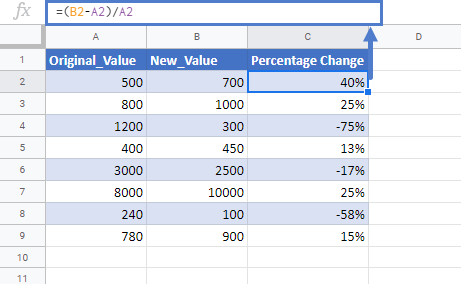# Calculate Percentage Change Between Numbers in Excel & Google Sheets

In this tutorial, we will learn how to create a calculate percentage change between two numbers in Excel & Google Sheets.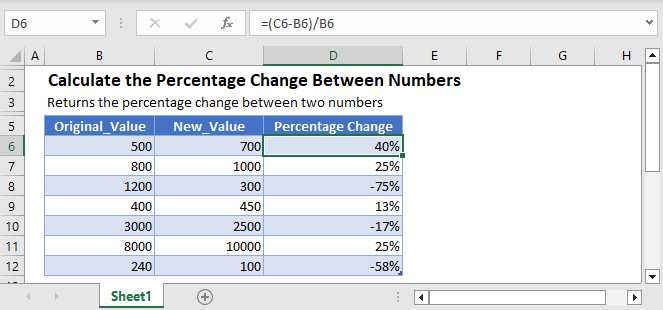## Percentage Change Formula

The percentage between the two values is simply calculated by taking the difference between two numbers and dividing it with the original number.

=(new_value– original_value)/original_value

For example, calculate the percentage change between the price of crude oil in April, i.e. \$20 per thousand barrels and its May price, i.e. \$30 per thousand barrels.

=(\$30-\$20)/\$20

=0.5

=50%

So, the crude oil price increased by 50% in May from the April price.

## Formula Breakdown

The Formula works simply by following the order of operations. The actual change is calculated first by subtracting the original_value from the new_value. After that, the difference is divided by the original_value.

That’s how we get the percentage change between two numbers

The formula can easily be understood by breaking it down into the following 4 simple steps:

### Step 1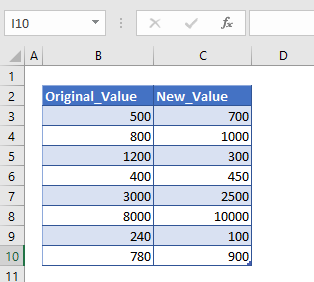First of all, we need to prepare the data for the calculation of percentage change. Here, in the above table, we’ve put some values that we are going to use to find the percentage difference between the orginal_values and new_values.

### Step 2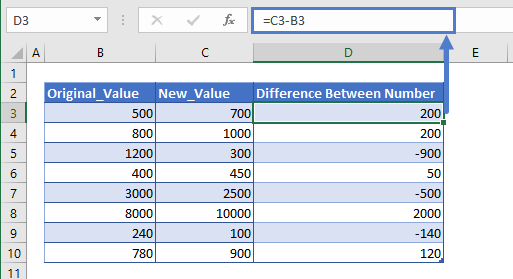After preparing the data, we’ll calculate the difference between the values. The difference is simply calculated by subtracting the column B values from column C values.

``=C3-B3``

The difference we calculated can have positive value or negative value, meaning the change in value can be positive and negative.

### Step 3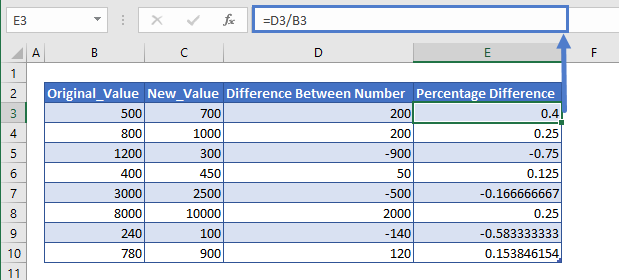Now we simply divide the difference value or change in value (column D) with the original_value (column B). This will give us the result in number format

``=D3/B3``

### Step 4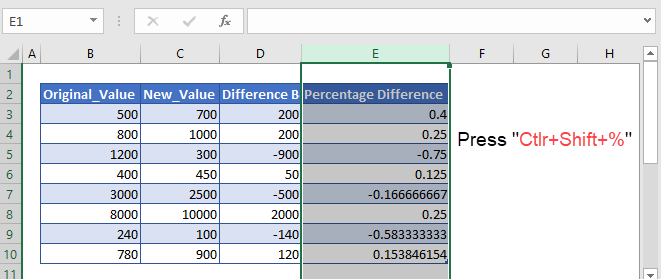The result we get in step 3 is in number format. We need the values in the percentage form. So, we’ll change the number format of the entire column E. To do so, first, we’ll select the entire column E and then press the shortcut key i.e. Ctrl+Shift+%, for changing the number format to Percent Style.

After this, we’ll get the percentage change between two numbers.

### Easy Excel Automation